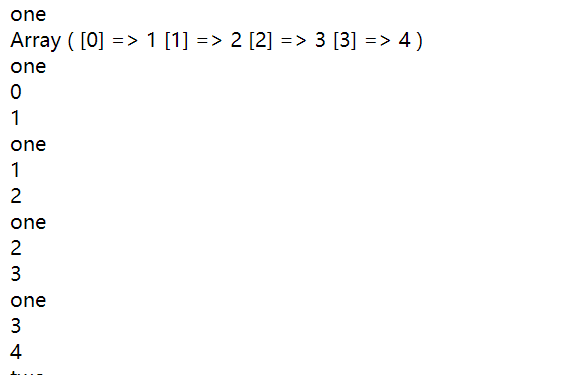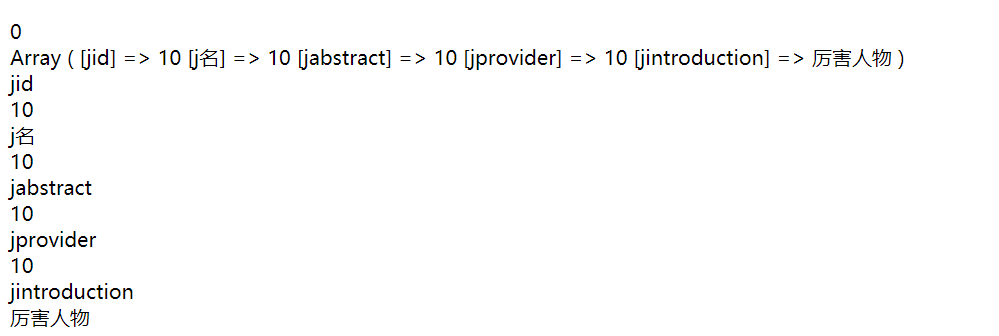# php中foreach循环遍历二维数组

（因为不知道该怎么设置where条件，设置只显示Jdate字段中今天以后的数据，所以只能在select查询后的数据中进行筛选..基础没大佬，真的挺麻烦）

foreach 语法结构提供了遍历数组的简单方式。foreach 仅能够应用于数组和对象，如果尝试应用于其他数据类型的变量，或者未初始化的变量将发出错误信息。有两种语法：

foreach (array_expression as $value) statement foreach (array_expression as$key => $value) statement  第一种格式遍历给定的 array_expression 数组。每次循环中，当前单元的值被赋给$value 并且数组内部的指针向前移一步（因此下一次循环中将会得到下一个单元）。

2     "one" => array(1,2,3,4),
3     "two" => array(5,6,7,8),
4     "three" => array('a','b','c','d')
5 );
6 foreach ($a as$k => $v) { 7 echo$k . '<br>';
8     print_r($v); 9 echo '<br>'; 10 foreach ($a[$k] as$index => $value) { 11 echo$k . '<br>';
12         echo $index . '<br>'; 13 echo$value . '<br>';
14     }
15 }$b=array ( '0' => Array ( 'jid' => 10,'j名' => 10,'jabstract' => 10,'jprovider' => 10,'jintroduction' => '厉害人物'), '1' => Array ( 'jid' => 8 ,'j名' => 8, 'jabstract' => 8, 'jprovider' => 8, 'jintroduction' => '厉害人物') ); 1 foreach ($b as $k =>$v) {
2     echo $k.'<br>'; 3 print_r($v);
4     echo '<br>';
5     foreach ($b[$k] as $index =>$value){
6         echo $index.'<br>'; 7 echo$value.'<br>';
8     }
9 }

输出跟上面的也是一样的原理2018-12-02

posted @ 2018-12-02 21:14  空眠  阅读(24107)  评论(0编辑  收藏  举报